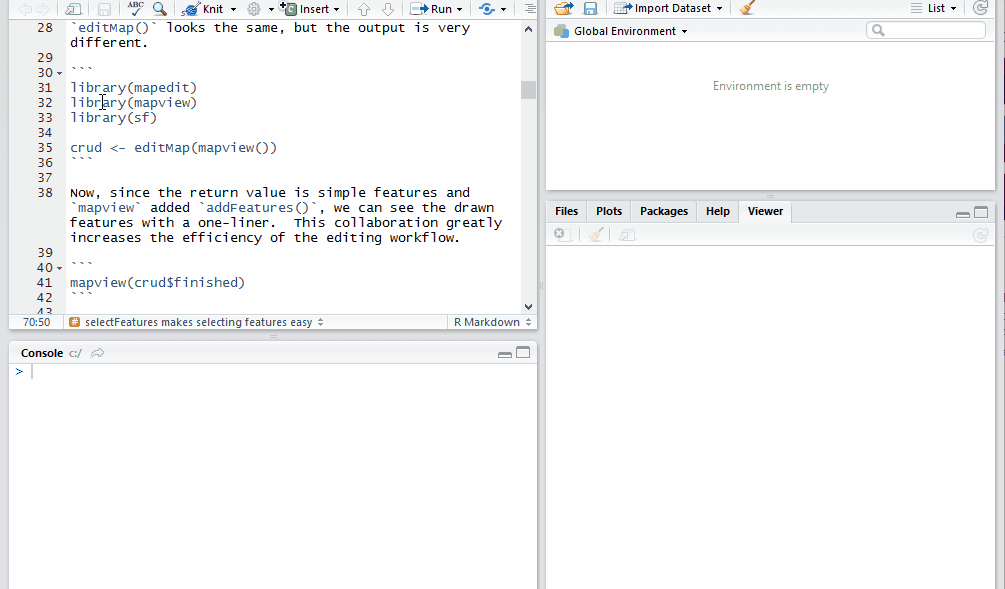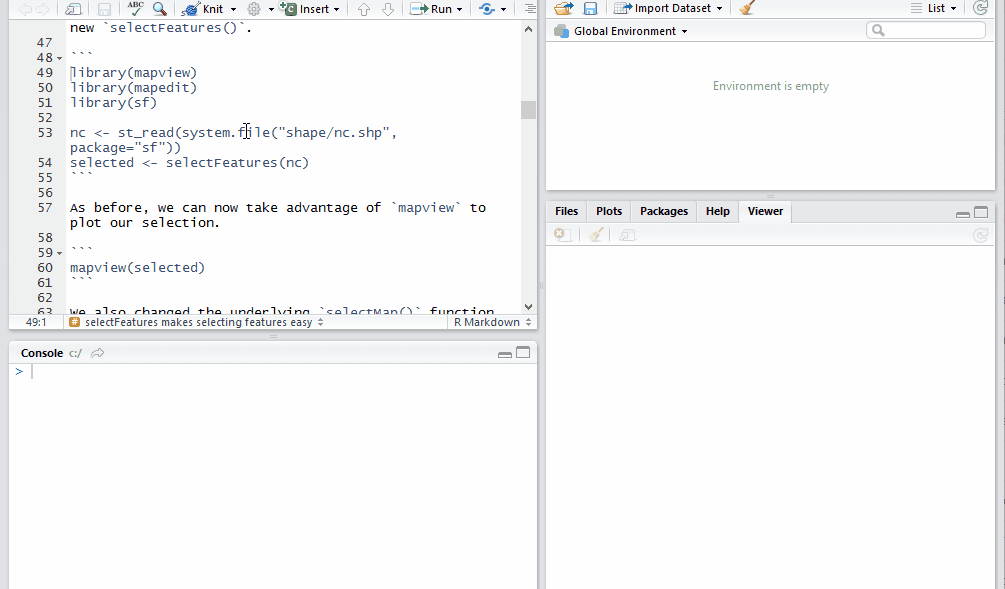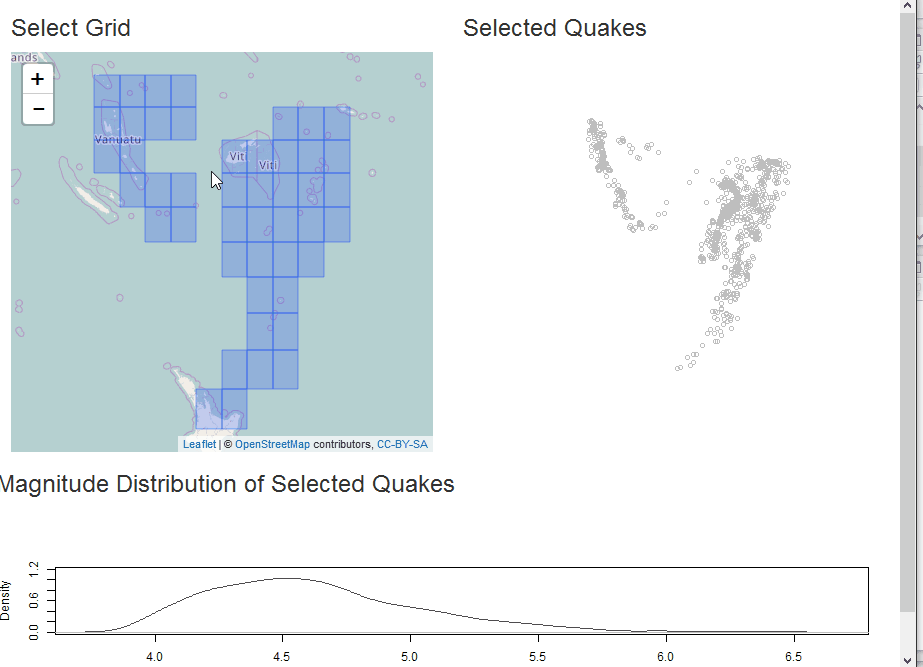`mapedit` has progressed substantially since the introduction to `mapedit` post. `mapedit 0.2.0` offers improvements and incorporates changes based on much appreciated feedback from the R geospatial community. `mapedit` is still in rapid development, but the API is stabilizing. We are targeting a CRAN release prior to useR 2017, and Tim Appelhans will demonstrate `mapedit` in his useR talk.

In this post, we will highlight some of the recent improvements and changes to `mapedit`. These updates can be categorized as

1. better integration with [simple features(https://github.com/edzer/sfr)] and

## Install

We are moving quickly, so please install the development versions of `mapview`, `leaflet.extras`, and `mapview` as shown below.

``````devtools::install_github("r-spatial/mapview@develop")
devtools::install_github("r-spatial/mapedit")
``````

## Simple Features

The R geo community is radidly embracing the RConsortium-sponsored `sf` package, and `mapedit` plans to fully adopt and incorporate simple features like `leaflet`, `mapview`, `geojsonio`, `plotly`, and `ggplot2`. `sf` can greatly improve geospatial workflows in R. `mapedit` now returns simple features by default with `editMap()` and includes a new function `selectFeatures()` for interactive selection of simple features. Let’s take a quick look at this new functionality.

### editMap returns sf

`editMap()` looks the same, but the output is very different.

``````library(mapedit)
library(mapview)
library(sf)

crud <- editMap(mapview())
``````

Now, since the return value is simple features and `mapview` added `addFeatures()`, we can see the drawn features with a one-liner. This collaboration greatly increases the efficiency of the editing workflow.

``````mapview(crud\$finished)
``````### selectFeatures makes selecting features easy

Let’s use the `sf` example with North Carolina county data to give us some simple features to select with the new `selectFeatures()`.

``````library(mapview)
library(mapedit)
library(sf)

selected <- selectFeatures(nc)
``````

As before, we can now take advantage of `mapview` to plot our selection.

``````mapview(selected)
``````We also changed the underlying `selectMap()` function to use the RStudio Viewer by default. This allows us to include `selectMap()` in a workflow or pipeline.

``````library(mapview)
library(mapedit)
library(sf)

selectFeatures(nc) %>%
st_union() %>%
mapview()
``````

Stay tuned for an `editFeatures()` equivalent.

## Shiny Modules

The original `editMap()` and `selectMap()` provided useful functionality. However, they are limited to standalone application. For even greater integration in an interactive geospatial workflow, Shiny modules allow a user to incorporate edit and select in a broader application context. Let’s see a couple examples of this concept.

### Select as Shiny Module

In this example, we will demonstrate analysis of the `quakes` data in `R` along with some helpful `sf`. The app will build a grid for selection of quakes and then plot the selection with a comparative density plot.

First we will convert the `quakes` to simple features.

``````library(sf)

# make the coordinates a numeric matrix
qk_mx <- data.matrix(quakes[,2:1])
# convert the coordinates to a multipoint feature
qk_mp <- st_multipoint(qk_mx)
# convert the multipoint feature to sf
qk_sf <- st_sf(st_cast(st_sfc(qk_mp), "POINT"), quakes, crs=4326)
``````

Now let’s use the very helpful `sf::st_make_grid()` function, and then filter the grid to only those that contain quakes points.

``````# make a grid
grd <- st_set_crs(st_make_grid(qk_sf), 4326)
# only keep grid polygons that contain at least one quake point
grd <- grd[which(sapply(st_contains(st_sf(grd), qk_sf),length)>0)]
``````

With our grid, we can build a Shiny app for some interactive analysis of quake magnitude.

``````library(mapview)
library(mapedit)
library(shiny)

ui <- fluidPage(
fluidRow(
column(
6,
h3("Select Grid"),
# our new select module ui
selectModUI("selectmap")
),
column(
6,
h3("Selected Quakes"),
plotOutput("selectplot")
)
),
fluidRow(
h3("Magnitude Distribution of Selected Quakes"),
plotOutput("quakestat", height=200)
)
)
server <- function(input, output, session) {
# our new select module
g_sel <- callModule(
selectMod,
"selectmap",
leaflet() %>%
)

rv <- reactiveValues(intersect=NULL, selectgrid=NULL)

observe({
# the select module returns a reactive
#   so let's use it to find the intersection
#   of selected grid with quakes points
gs <- g_sel()
rv\$selectgrid <- st_sf(
grd[as.numeric(gs[which(gs\$selected==TRUE),"id"])]
)
if(length(rv\$selectgrid) > 0) {
rv\$intersect <- st_intersection(rv\$selectgrid, qk_sf)
} else {
rv\$intersect <- NULL
}
})

output\$selectplot <- renderPlot({
plot(qk_mp, col="gray")
if(!is.null(rv\$intersect)) {
}
})

output\$quakestat <- renderPlot({
plot(
stats::density(qk_sf\$mag), col="gray30", ylim=c(0,1.2),
main = NA
)
if(!is.null(rv\$intersect) && nrow(rv\$intersect) > 0) {
lines(stats::density(rv\$intersect\$mag), col="red", lwd=2)
}
})
}
shinyApp(ui, server)
``````### Edit as Shiny Module

Since we have the quake data, we will use it to show the edit module in a simple application. Instead of the grid, let’s draw polygons to select quakes.

``````# run select demo for the quake data
#  we will need the qk_sf
#  to test
# plot(qk_sf)

library(mapedit)
library(mapview)
library(shiny)

ui <- fluidPage(
fluidRow(
# edit module ui
column(6, editModUI("editor")),
column(
6,
h3("Boxplot of Depth"),
plotOutput("selectstat")
)
)
)
server <- function(input, output, session) {
# edit module returns sf
edits <- callModule(editMod, "editor", mapview(qk_sf)@map)

output\$selectstat <- renderPlot({
req(edits()\$finished)
qk_intersect <- st_intersection(edits()\$finished, qk_sf)
req(nrow(qk_intersect) > 0)
boxplot(
list(
all = as.numeric(qk_sf\$depth),
selected = as.numeric(qk_intersect\$depth)
),
xlab = "depth"
)
})
}
shinyApp(ui, server)
``````## Next Steps

The progress made thus far depended entirely on feedback received. Please help us by providing feedback, ideas, and use cases. As mentioned earlier, we aim for an initial CRAN release before useR 2017 on July 4, 2017. We do not anticipate any breaking API changes before release. Rather, we plan to spend time on documentation, examples, and tests.

## RConsortium

`mapedit` and many of its dependency packages are funded by the RConsortium. Thanks so much to all those who have contributed to this fantastic organization. Also, thanks to all those open source contributors in the R community.